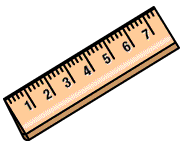### Measurement

```
LENGTH AND PERIMETERBIG IDEAS:

(taken from “Big Ideas by Dr. Small”):

There is always value in estimating a measurement.
The length of an object is a one-dimensional attribute. Length can be the measurement of a single measure of an object or a combined linear measure, like perimeter.

STUDENT LEARNING GOALS:

GOAL: I can convert metric units between kilometres, metres, centimetres and millimetres.

QUIZ: Metric Relationships (converting units)
VIDEO: Metric Conversion (this is not a Khan video but does outline some algorithms around metric conversions)
GAME: Metric Matching
GAME: Metric System Rockets
GAME: Metric Measurement Millionaire

GOAL: I can use a ruler to measure to nearest tenth of centimeter (i.e. mm).

QUIZ: Measuring Lengths
QUIZ: Measuring Perimeter
QUIZ: Perimeter of Rectangles
VIDEO: Comparing Lengths (1 video, 1 practice quiz)
VIDEO: Estimating Lengths (1 video, 1 practice quiz)
VIDEO: Measurement Word Problems (1 video, 1 practice quiz)

CURRICULUM EXPECTATIONS:

select and justify the most appropriate metric unit (i.e., millimetre, centimetre, decimetre, metre, decametre, kilometre) to measure length or distance in a given real-life situation.
Solve problems requiring conversion from larger to smaller metric units (eg., metres to centimetres, kilograms to grams, litres to millilitres)

AREA, Surface Area, PERIMETER AND VOLUMEUNIT: Area and Volume

BIG IDEAS (taken from “Big Ideas by Dr. Small”):

The length of an object is a one-dimensional attribute. Length can be the measurement of a single measure of an object or a combined linear measure, like perimeter.

The area of an object is a two-dimensional attribute. Area can be a single measure of a 2-D shape on an object or a combined measure of a 3-D shape, like surface area.
The volume of a 3-D object tells how much material it takes to build the object and the capacity of an object tells how much it will hold.

STUDENT LEARNING GOALS:

I can determine the area and perimeter of a rectangle
I can create a rectangle given the area and/or perimeter
I can determine the volume of a rectangular prism.

CURRICULUM EXPECTATIONS:

construct a rectangle, a square, a triangle, and a parallelogram using a variety of tools, given the area and/or perimeter.
determine, through investigation, the relationship between the area of a rectangle and the areas of parallelograms and triangles, by decomposing and composing
develop the formulas for the area of a parallelogram and the area of a triangle
solve problems involving the estimation and calculation of the areas of triangles and the areas of parallelograms
determine, using concrete materials, the relationship between units use to measure area and apply the relationship to solve problems that involve conversions from square metres to square centimetres.
determine, through investigation using a variety of tools and strategies, the relationship between the height, the area of the base, and the volume of a triangular prism and generalize to develop the formula.
determine through investigation using a variety of tools and strategies, the surface area of a rectangular and triangular prisms.
Solve problems involving the estimation and calculation of the surface area and volume of triangular and rectangular prisms.

Area of Polygons
Relating Perimeter and Area of Rectangles
Area Rule for Rectangles
Modelling Area
Measuring and Comparing Capacity
Measuring and Comparing Volume
Volume versus Capacity

Introduction to Perimeter
Perimeter of a Shape
Introduction to Area and Square Units
Rectangle Area
Comparing Perimeter and Area of Rectangles
Finding Length and Width if the Area/Perimeter is Known
Finding Unknown Dimensions
Volume: How to Measure it
Calculating Volume using a Formula
Volume Word Problems

ONLINE GAMES:

Perimeter and Area (Rectangles)

```